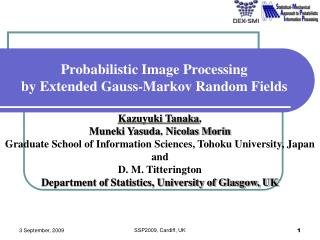DownloadDownload PresentationProbabilistic Image Processing by Extended Gauss-Markov Random Fields

# Probabilistic Image Processing by Extended Gauss-Markov Random Fields

Download Presentation## Probabilistic Image Processing by Extended Gauss-Markov Random Fields

- - - - - - - - - - - - - - - - - - - - - - - - - - - E N D - - - - - - - - - - - - - - - - - - - - - - - - - - -
##### Presentation Transcript

1. Probabilistic Image Processingby Extended Gauss-Markov Random Fields Kazuyuki Tanaka, Muneki Yasuda, Nicolas Morin Graduate School of Information Sciences, Tohoku University, Japan and D. M. Titterington Department of Statistics, University of Glasgow, UK SSP2009, Cardiff, UK

2. Noise Assumption 2: Degraded images are randomly generated from the original image by according to the conditional probability of degradation process. Image Restoration by Bayesian Statistics Transmission Posterior Original Image Degraded Image Estimate Assumption 1: Original images are randomly generated by according to a prior probability. Bayes Formula SSP2009, Cardiff, UK

3. Assumption 1: Prior Probability consists of a product of functions defined on the neighbouring pixels. Bayesian Image Analysis Prior Probability Gibbs Sampler SSP2009, Cardiff, UK

4. Bayesian Image Analysis Assumption 2: Degraded image is generated from the original image by Additive White Gaussian Noise. V:Set of all the pixels SSP2009, Cardiff, UK

5. Bayesian Image Analysis Degraded Image Original Image Degradation Process Prior Probability Posterior Probability Estimate Bayesian Network Data Dominant Smoothing SSP2009, Cardiff, UK

6. Average of the posterior probability can be calculated by using the multi-dimensional Gauss integral Formula Average of Posterior Probability Gaussian Integral formula SSP2009, Cardiff, UK

7. Hyperparameters a, s are determinedso as to maximize the marginal likelihood Pr{Y=y|a,g,s} with respect to a, s. Statistical Estimation of Hyperparameters Original Image Degraded Image Marginalized with respect to X Marginal Likelihood SSP2009, Cardiff, UK

8. Statistical Estimation of Hyperparameters Gaussian Integral formula SSP2009, Cardiff, UK

9. Exact Expression of Marginal Likelihood in Gaussian Graphical Model Extremum Conditions for a and s Iterated Algorithm EM Algorithm SSP2009, Cardiff, UK

10. Iteration Procedure of EM Algorithm in Gaussian Graphical Model Bayesian Image Analysis by Gaussian Graphical Model EM SSP2009, Cardiff, UK

11. Image Restoration by Gaussian Markov Random Field (GMRF) Model and Conventional Filters Original Image Degraded Image Restored Image Conventional GMRF g=0 Extended GMRF g=-0.45 Extended GMRF g=-0.2 Simultaneous AR (0.00088,33) (0.00465,36) (0.00171,38) SSP2009, Cardiff, UK

12. Image Restoration by Gaussian Markov Random Field (GMRF) Model and Conventional Filters Original Image Degraded Image Restored Image Conventional GMRF g=0 Extended GMRF g=-0.45 Simultaneous AR Extended GMRF g=-0.2 (0.00123,39) (0.00687,41) (0.00400,43) SSP2009, Cardiff, UK

13. Statistical Performance by Sample Average of Numerical Experiments Sample Average of Mean Square Error Noise Posterior Probability Original Images Degraded Images Restored Images SSP2009, Cardiff, UK

14. Statistical Performance Estimation Degraded Image Original Image Additive White Gaussian Noise Restored Image Additive White Gaussian Noise Posterior Probability SSP2009, Cardiff, UK

15. Statistical Performance Estimation for Gauss Markov Random Fields = 0 SSP2009, Cardiff, UK

16. Statistical Performance Estimation for Gauss Markov Random Fields s=40 s=40 g=0 g=0 g=-0.2 g=-0.2 g=-0.45 g=-0.45 a a SSP2009, Cardiff, UK

17. Summary • We propose an extension of the Gauss-Markov random field models byintroducing next-nearest neighbour interactions. Values for the hyperparameters in the proposed model are determined by using the EM algorithm in order to maximize the marginal likelihood. • In addition, a measure of mean squared error, which quantifies the statistical performance of our proposed model, is derived analytically as an exact explicit expression by means of the multi-dimensional Gaussian integral formulas. Statistical performance analysis of probabilistic image processing for our extended Gauss Markov Random Fields has been shown. SSP2009, Cardiff, UK

18. References • K. Tanaka: Statistical-mechanical approach to image processing (Topical Review), Journal of Physics A: Mathematical and General, vol.35, no.37, pp.R81-R150, 2002. • K. Tanaka and J. Inoue: Maximum Likelihood Hyperparameter Estimation for Solvable Markov Random Field Model in Image Restoration, IEICE Transactions on Information and Systems, vol.E85-D, no.3, pp.546-557, 2002. • K. Tanaka, J. Inoue and D. M. Titterington: Probabilistic Image Processing by Means of Bethe Approximation for Q-Ising Model, Journal of Physics A: Mathematical and General, vol. 36, no. 43, pp.11023-11036, 2003. • K. Tanaka, H. Shouno, M. Okada and D. M. Titterington: Accuracy of the Bethe Approximation for Hyperparameter Estimation in Probabilistic Image Processing, Journal of Physics A: Mathematical and General, vol.37, no.36, pp.8675-8696, 2004. • K. Tanaka and D. M. Titterington: Statistical Trajectory of Approximate EM Algorithm for Probabilistic Image Processing, Journal of Physics A: Mathematical and Theoretical, vol.40, no.37, pp.11285-11300, 2007. SSP2009, Cardiff, UK